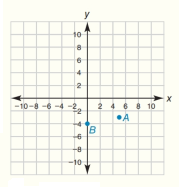Chapter 10.CT, Problem 4CTElementary Geometry For College St...

7th Edition
Alexander + 2 others
ISBN: 9781337614085

Solutions

Chapter
SectionElementary Geometry For College St...

7th Edition
Alexander + 2 others
ISBN: 9781337614085
Textbook Problem

In the form ( x , y ) , determine the midpoint of C D - as described in Exercise 2.In the coordinate system for Exercise 1, plot and label each point: C ( - 6 ,   1 ) and D ( 0 ,   9 )To determine

To find:

The midpoint of the line CD- using midpoint formula where the coordinates are C(-6, 1) and D0, 9.

Explanation

Given points are,

C(-6, 1) and D(0, 9)

Point C is located at a distance from origin in the x-axis =-6

Point C is located at a distance from origin in the y-axis =1

Similarly, point D is located at a distance from origin in the x-axis =0

Point D is located at a distance from origin in the y-axis =9

Sketch the given points in graph and name them as C and D and joined the points as shown in the figure,

Formula for the midpoint is, xm,ym=x1+x22,y1+y22

Still sussing out bartleby?

Check out a sample textbook solution.

See a sample solution

The Solution to Your Study Problems

Bartleby provides explanations to thousands of textbook problems written by our experts, many with advanced degrees!

Get Started

Evaluate the indefinite integral. eu(1eu)2du

Single Variable Calculus: Early Transcendentals, Volume I

In Exercises 2932, find the value(s) of x that satisfy the expression. 29. 2x2 + 3x 2 0

Applied Calculus for the Managerial, Life, and Social Sciences: A Brief Approach

Expand each expression in Exercises 122. (3x+1)2

Finite Mathematics and Applied Calculus (MindTap Course List)

It does not exist.

Study Guide for Stewart's Multivariable Calculus, 8th